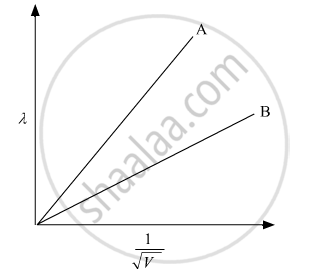# Plot a Graph Showing Variation of De Broglie Wavelength Versus Accelerating Potential - Physics

Plot a graph showing variation of de Broglie wavelength λ versus 1/sqrtV , where V is accelerating potential for two particles A and B, carrying the same charge but different masses m1, m2 (m1 > m2). Which one of the two represents a particle of smaller mass and why?

#### Solution

Given: Charge (q) is the same but masses of both the particles are different (mm2).

The de Brogile wavelength given by

lambda = h/sqrt(2mqV)

The slope of the graph λ versus 1/sqrtV is h/sqrt(2mq)The slope of the smaller mass is larger; therefore, plot A in the above graph is for mass m2

Concept: de-Broglie Relation
Is there an error in this question or solution?
2015-2016 (March) Delhi Set 1

Share